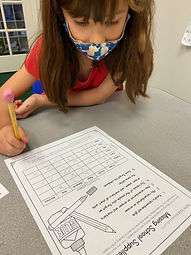## Ms. Brianne

### Target 1​

###### Lesson Type:

Continuation

Number Operation

:

Fractional Numbers

Understand that a fraction is a quantity formed when a whole is partitioned into equal parts.

###### 1:

Compose a written fraction to describe a visual or a visual to describe a written fraction.

###### 2:

Identify the number of shaded or unshaded parts as the numerator of a fraction.

###### 3:

Identify the number of equal parts as the denominator of a fraction.

###### 4:

Understand and explain what a fraction is.

3rd

###### Vocabulary:

Fraction, Whole, Parts, Equal, Numerator, Denominator

Activities:

• Students drew basic shapes and cut it into a specific amount of equal parts.
• Students learned the numerator is how many equal parts they have.
• Students drew pictorial representations on a whiteboard to show fractions.
• Students played a fraction bingo game. They rolled 2 dice and used them for the numerator and denominator in a fraction. They had to find the circle with the matching denominator and color in the matching numerator to try and get 5 in a row.### Home Exploration

###### Guiding Questions:## Absent Students:

### Target 2

:

###### 1:

Study a given arithmetic sequence to determine what rule explains the sequence (i.e., 2-4-6-8-10…each number is the sequence gets bigger by 2).

###### 2:

Write an arithmetic rule to explain a given sequence.

###### 3:

Understand that a pattern can be described by stating the rule that it is following.

3rd

###### Vocabulary:

Pattern, Sequence

Activities:

• Students figured out the pattern in a sequence of numbers and determined the next few numbers in the sequence. Then they wrote the rule for the arithmetic sequence.
• Students used reasoning to help figure out the pattern rule. For example, if the numbers are increasing, the expression most likely deals with addition or multiplication. But if the numbers are increasing by a constant number, it involves addition.### Home Exploration

###### Guiding Questions:### Target 3

:

###### 1:

Use the given information in a problem to identify the correct answer.

###### 2:

Use the given information to determine which is not the correct answer.

4th

###### Vocabulary:

Logic, Reasoning, Clues

Activities:

• Students were given a word problem in which they used given clues and the process of elimination to find the item each person was missing.
• Students used a X if they figured out it was not possible, and an O if they determined it was the missing item.### Home Exploration